# Beginning Algebra Tutorial 24

Beginning Algebra
Tutorial 24: Graphing Linear InequalitiesLearning Objectives

After completing this tutorial, you should be able to:
1. Graph linear inequalities in two variables.Introduction

In this tutorial we will be looking at linear inequalities in two variables.  It will start out exactly the same as graphing linear equations and then we get to color in the region of the coordinate system that correlates with the inequality.  Some of these problems may get a little long.  Don't let that discourage you, you can do it. Hang in there, a lot of the steps are concepts from the past, things you should already have seen and done before.  I will put in links to the material that you need to remember from the past, in case you need a review.   A lot of times math works that way, use what you know to learn the new concept.  Let's see what you can do with these inequalities.Tutorial

Linear Inequalities in Two Variables

A linear inequality in two variables is any expression
that can be put in the form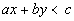where a, b, and c are constants

Note that the above definition can be applied to any of the following four inequalities: <, >, <, or >.

The solution set and graph for a linear inequality is a region of the rectangular coordinate system.  Recall that the graph of a linear equation is a straight line.  The inequality sign extends this to being on one side of the line or the other on the graph.

Graphing a Linear Inequality

Step 1:  Graph the boundary line.

Essentially you graph the boundary line the same as if the problem was a linear equation.  Pretend that there is an equal sign and use an appropriate method to graph the line.  Unless the directions to a problem indicate otherwise, you can use any method to graph it.  The ways covered in our past tutorials are plotting any three points as shown in Tutorial 21: Graphing Linear Equations or using the x and y intercepts as shown in Tutorial 22: Intercepts.

When you draw the boundary line, you need to have a way to indicate if the line is included or not in the final answer.

Solid boundary line: < or >
If the problem includes where it is equal, then you will have a solid boundary line.  In other words, if you have < or > , you will have a solid line for your boundary line.

This shows the boundary line for x + y < 6:
(note that this does not show the inequality part)Dashed boundary line: < or >
If the problem does not include where it is equal, then you will use a dashed boundary line.  In other words, if you have < or >, you will have a dashed line for your boundary line.

This shows the boundary line for x + y < 6:
(note that this does not show the inequality part)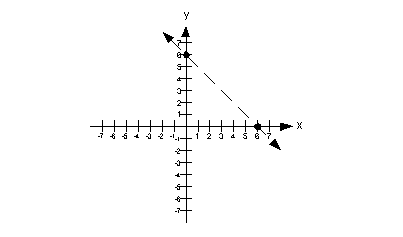In either case, you still graph the line the same.  You just have to decide if you are needing a solid line or a dashed line.

The boundary line separates the rectangular coordinate system into two parts.  One of those parts will make the inequality true and be it’s solution.

Step 2:  Plug in a test point that is not on the boundary line.

Pick a test point on either side of the boundary line and plug it into the original problem.  This will help determine which side of the boundary line is the solution.

If you get a true statement when you plug in the test point in step 2, then you have found a solution.  Shade the region that the test point is in

If you get a false statement when you plug in the test point in step 2, then you don’t have a solution.  Shade in the region that is on the other side of the test point.

It doesn’t matter what you use for the test point as long as it is not on the
boundary line.  You want to keep it as simple as possible.Example 1:   Graph the inequality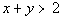.

Step 1:  Graph the boundary line.

I’m going to use the intercepts to help me graph the boundary line.  Again, you can use any method that you want, unless the directions say otherwise.

When I’m working with only the boundary line, I will put an equal sign between the two sides to emphasize that we are working on the boundary line.  That doesn’t mean that I changed the problem. When we put it all together in the end, I will put the inequality back in.

What value is y on the x-intercept?
If you said 0, you are correct.
If you need a review on x-intercepts, go to Tutorial 22: Intercepts.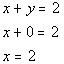*Replace y with 0
*x-intercept

x-intercept is (2, 0)

What is the value of x on the y-intercept?
If you said 0, you are correct.
If you need a review on y-intercepts, go to Tutorial 22: Intercepts.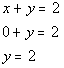*Replace x with 0
*y-intercept

y-intercept is (0, 2).

Plug in 1 for x to get a third solution: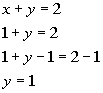*Replace x with 1
*Inverse of add 1 is sub. 1

(1, 1) is another solution on the boundary line.

Solutions:

x y (x, y) 2 0 (2, 0) 0 2 (0, 2) 1 1 (1, 1)

Since the original problem has a >, this means it DOES NOT include the boundary line.

So are we going to draw a solid or a dashed line for this problem?
It looks like it will have to be a dashed line.

Putting it all together, we get the following boundary line for this problem: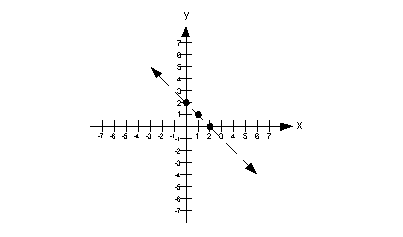Step 2:  Plug in a test point that is not on the boundary line.

Note how the boundary line separates it into two parts.

An easy test point would be (0, 0).  Note that it is a point that is not on the boundary line. In fact, it is located below the boundary line.

Let’s put (0, 0) into the original problem and see what happens: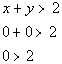*Replacing x and y with 0
*False statement

Since our test point (0, 0) made our inequality FALSE, this means it is not a solution.

Since it has to be on one side or the other of the boundary line, and it is not below it, then our solution would lie above the boundary line.  This means we will shade in the part that is above it.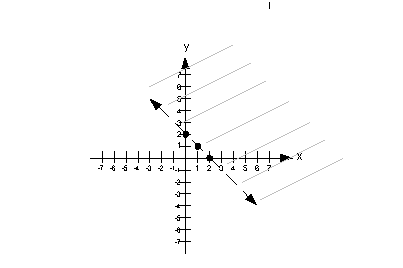Example 2:   Graph the inequality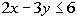.

Step 1:  Graph the boundary line.

I’m going to use the intercepts to help me graph the boundary line.  Again, you can use any method that you want, unless the directions say otherwise.

When I’m working with only the boundary line, I will put an equal sign between the two sides to emphasize that we are working on the boundary line.  That doesn’t mean that I changed the problem. When we put it all together in the end, I will put the inequality back in.

What value is y on the x-intercept?
If you said 0, you are correct.
If you need a review on x-intercepts, go to Tutorial 22: Intercepts.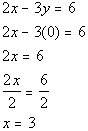*Replace y with 0

*Inverse of mult. by 2 is div. by 2

*x-intercept

x-intercept is (3, 0)

What is the value of x on the y-intercept?
If you said 0, you are correct.
If you need a review on y-intercepts, go to Tutorial 22: Intercepts.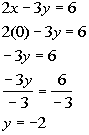*Replace x with 0

*Inverse of mult. by -3 is div. by -3

*y-intercept

y-intercept is (0, -2).

Plug in 1 for x to get a third solution: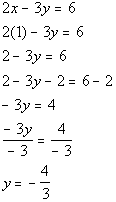*Replace x with 1

*Inverse of add 2 is sub. 2

*Inverse of mult. by -3 is div. by -3

(1, -4/3) is another solution on the boundary line.

Solutions:

x y (x, y) 3 0 (3, 0) 0 -2 (0, -2) 1 -4/3 (1, -4/3)

Since the original problem has a <, this means it DOES  include the boundary line.

So are we going to draw a solid or a dashed line for this problem?
It looks like it will have to be a solid line.

Putting it all together, we get the following boundary line for this problem: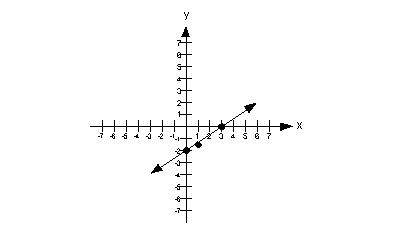Step 2:  Plug in a test point that is not on the boundary line.

Note how the boundary line separates it into two parts.

An easy test point would be (0, 0).  Note that it is a point that is not on the boundary line. In fact, it is located above the boundary line.

Let’s put (0, 0) into the original problem and see what happens: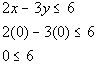*Replace x and y with 0
*True statement

Since our test point (0, 0) made our inequality TRUE, this means it is a solution.

Our solution would lie above the boundary line.  This means we will shade in the part that is above it.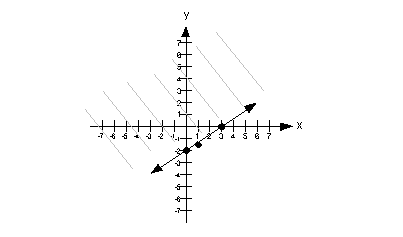Example 3:   Graph the inequality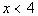.

Step 1:  Graph the boundary line.

If we wrote this as an equation, it would be x = 4.  This is in the form x = c, which is one of our “special” lines.

Do you remember what type of line x = c graphs as?
It comes out to be a vertical line.
If you need a review on vertical lines, go to Tutorial 22: Intercepts

Every x’s value on the boundary line would have to be 4.

Solutions:

x y (x, y) 4 0 (4, 0) 4 1 (4, 1) 4 2 (4, 2)

Since the original problem has a <, this means it DOES NOT include the boundary line.

So are we going to draw a solid or a dashed line for this problem?
It looks like it will have to be a dashed line.

Putting it all together, we get the following boundary line for this problem: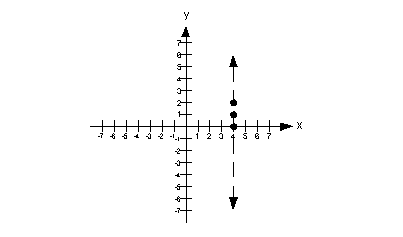Step 2:  Plug in a test point that is not on the boundary line.

Note how the boundary line separates it into two parts.

An easy test point would be (0, 0).  Note that it is a point that is not on the boundary line. In fact, it is located to the left of the boundary line.

Let’s put (0, 0) into the original problem and see what happens: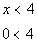*Replace x with 0
*True Statement

Since our test point (0, 0) made our inequality TRUE, this means it is a solution.

Our solution would lie to the left of the boundary line.  This means we will shade in the part that is to the left of it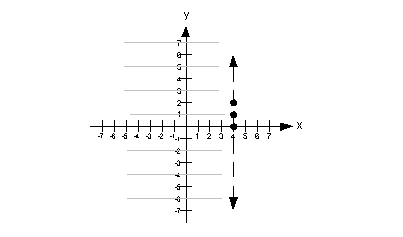Practice Problems

Ā
These are practice problems to help bring you to the next level.  It will allow you to check and see if you have an understanding of these types of problems. Math works just like anything else, if you want to get good at it, then you need to practice it.  Even the best athletes and musicians had help along the way and lots of practice, practice, practice, to get good at their sport or instrument.  In fact there is no such thing as too much practice.

To get the most out of these, you should work the problem out on your own and then check your answer by clicking on the link for the answer/discussion for that  problem.  At the link you will find the answer as well as any steps that went into finding that answer.Practice Problems 1a - 1b: Graph each inequality.

1a.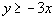1b.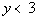Need Extra Help on these Topics?

The following is a webpage that can assist you in the topics that were covered on this page:

http://www.purplemath.com/modules/ineqgrph.htm
This website helps you with graphing linear inequalities.

Go to Get Help Outside the Classroom found in Tutorial 1: How to Succeed in a Math Class for some more suggestions.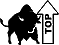Last revised on July 31, 2011 by Kim Seward.